# Lorenz equations demo

Simulate the Lorenz equations twice, with two initial conditions that are small perturbations of fixed point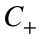, as defined in Strogatz. Plot the first component of each solution over time.
sigma=10; b=8/3; r=28;
f=@(t,y)lorenzODE(t,y,sigma,r,b);
fixedPoint=[sqrt(b*(r-1)),sqrt(b*(r-1)),r-1];
perturbation=[1e-2 0 0];
y01=fixedPoint+perturbation;
y02=fixedPoint-perturbation;
tFinal=75;
dt=0.1;
tspan=0:dt:tFinal; %Note that by specifying tspan with more than two elements, this gives the output array t
[t1,y1]=ode45(f,tspan,y01);
[t2,y2]=ode45(f,tspan,y02);
plot(t1,y1(:,1),t2,y2(:,1))
xlabel('$t$'); ylabel('$x$')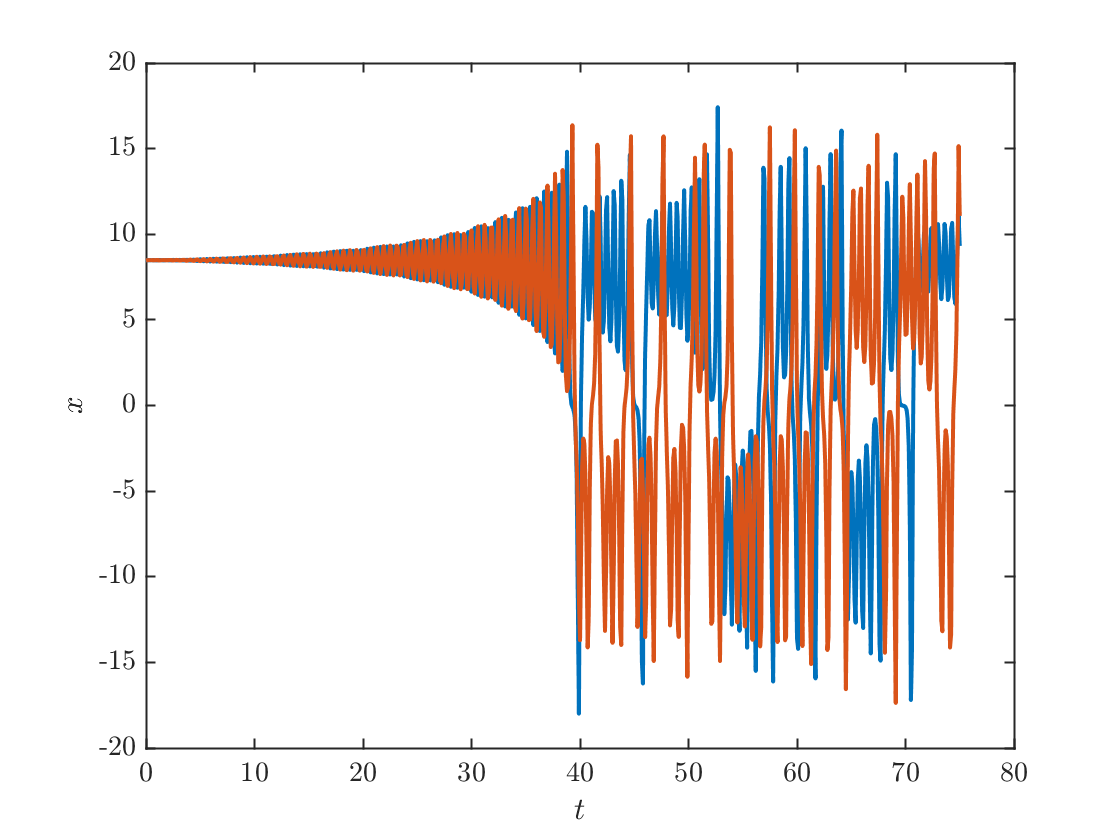Subtract the two solutions to create yDiff. Then compute the length of yDiff at each time, to find yDistance. The distance grows exponentially, which we can see by plotting the distance in logarithmic scale on the y-axis and linear scale on the x-axis using the command semilogy.
yDiff=y1-y2;
yDistance=sqrt(sum(yDiff.^2,2));
clf;
semilogy(t1,yDist)
xlabel('$t$');ylabel('$\|\Delta \vec{x} \|$')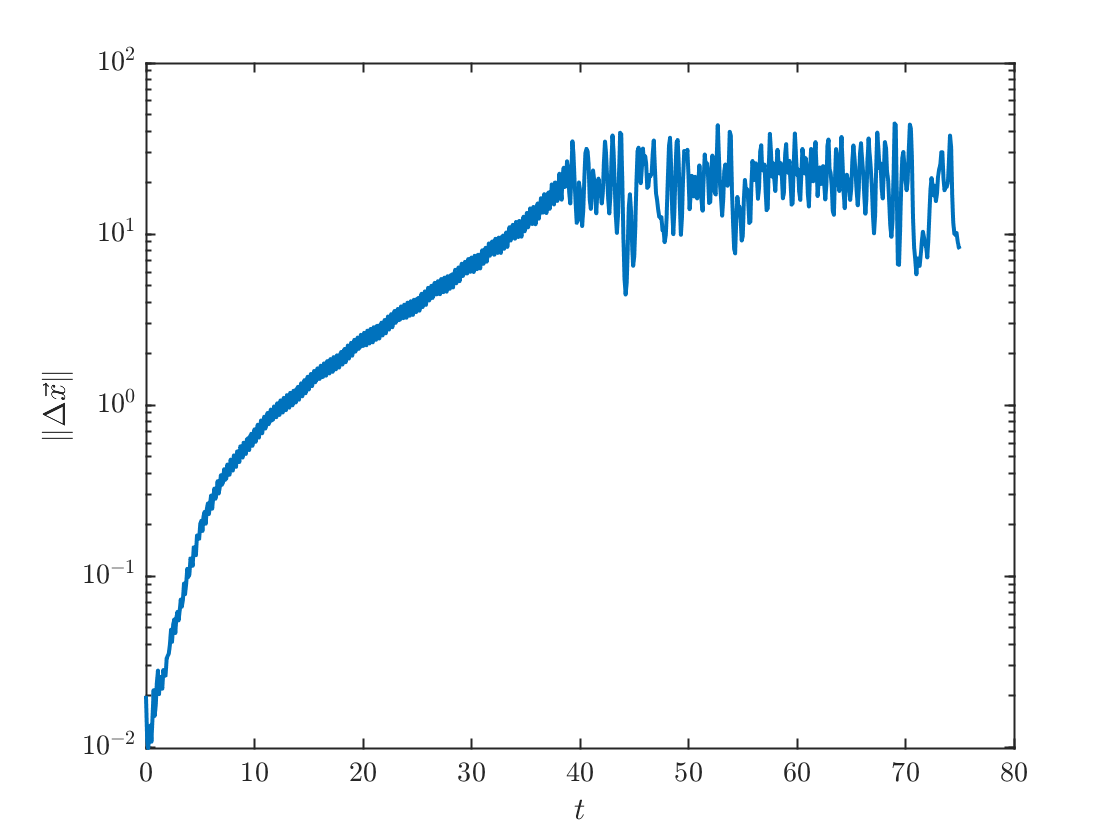## Constructing the Lorenz map

To construct the Lorenz map, we need to locate the local maxima of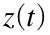. To do this we use the Events Location feature in the matlab ODE solver. We have to create an event function. At a local maximum of, we must haveand in particular it must go through zero from positive to negative.
fEvent=@(t,y)lorenzMaxEvent(t,y,b);
options=odeset('events',fEvent);
[t1,y1,te,ye,ie]=ode45(f,[0 tFinal],y01,options);
plot(t1,y1,te,ye(:,3),'o');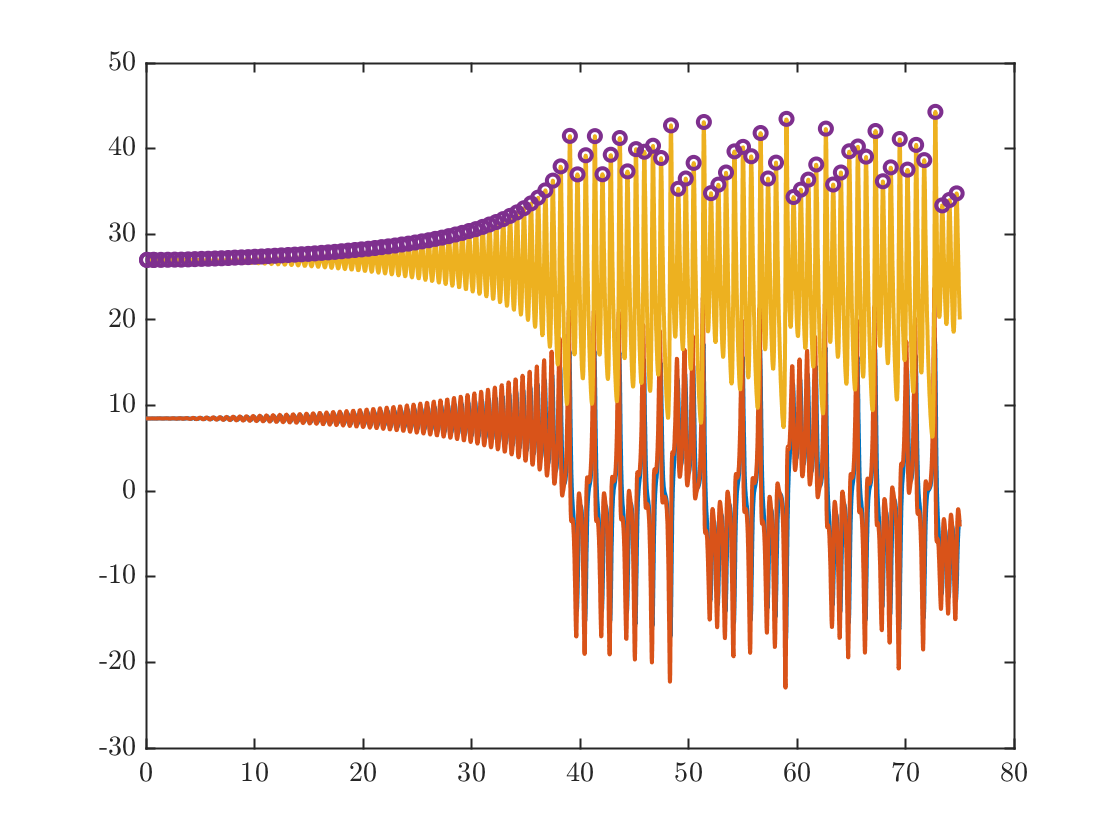xlabel('$t$');
ylabel('$x,y,z$')
We note from the above plot that on the attractor the motion of x and y is highly correlated, so instead of plotting the 3d trajectories, we just plot x and z components;
plot(y1(:,1),y1(:,3),ye(:,1),ye(:,3),'o')
xlabel('$x$');ylabel('$z$')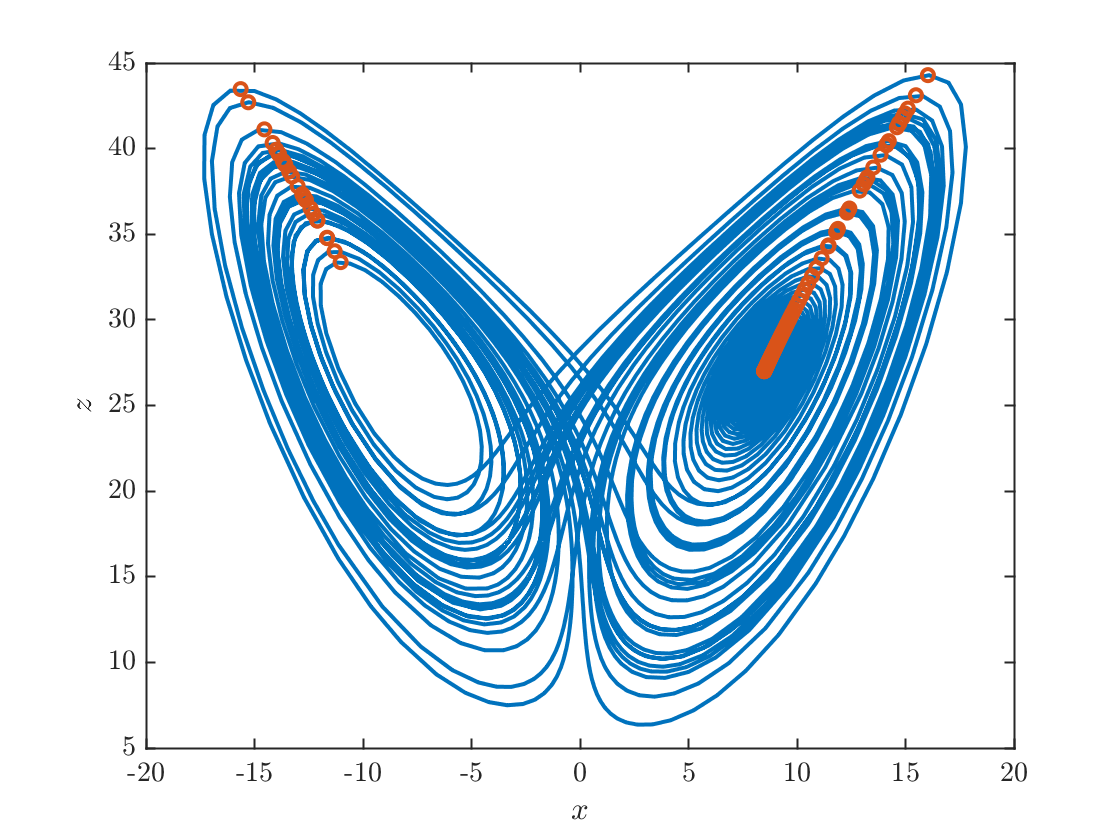### Plotting the Lorenz map

Now we plot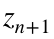as a function of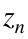. We also plot the diagonal line for comparison.
z=ye(:,3);
zmin=min(z);zmax=max(z);
plot(z(1:end-1),z(2:end),'o',[zmin zmax],[zmin zmax])
axis equal
xlabel('$z_{n}$');ylabel('$z_{n+1}$')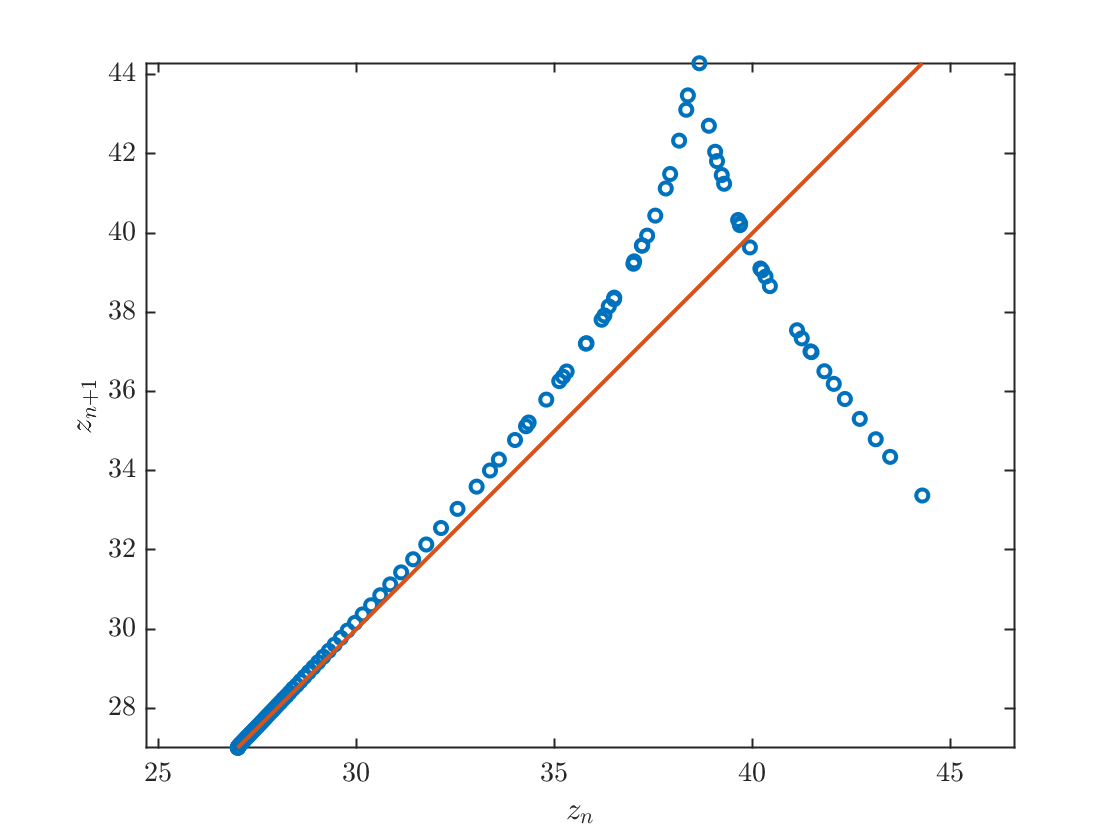## Function definitions

#### The ordinary differential equation

function dydt= lorenzODE(~,y,sigma,r,b)
dydt=zeros(3,1);
dydt(1) = sigma*(y(2)-y(1));
dydt(2) = r*y(1)-y(2) - y(1)*y(3);
dydt(3)= y(1)*y(2)-b*y(3);
end

#### The events locator

function [value, isterminal,direction]=lorenzMaxEvent(~,y,b)
value=y(1)*y(2)-b*y(3); % This sets dz/dt=0
isterminal=0; % If isterminal=1, then the simulation quits when the event occurs
direction = -1; % because we want the event to cross zero in a decreasing direction